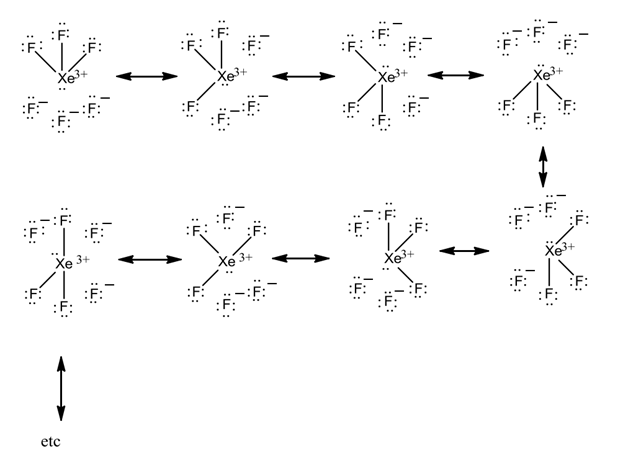# Drawing $$XeF_6$$ without an expanded octet
Most descriptions of the bonding in $$XeF_6$$ invoke an "expanded octet" model. However, an expanded octet is not required to draw \XeF_6\). Alternatively, the structure of $$XeF_6$$ can be described as a resonance hybrid of octet-conforming structures, as shown below.The calculated Mullkin charge at the xenon in $$XeF_6$$ is approximately +2.3, which is very similar to the charge of +3 that is predicted based on this resonance model, and suggests that "expanded octet" is not an entirely valid model.